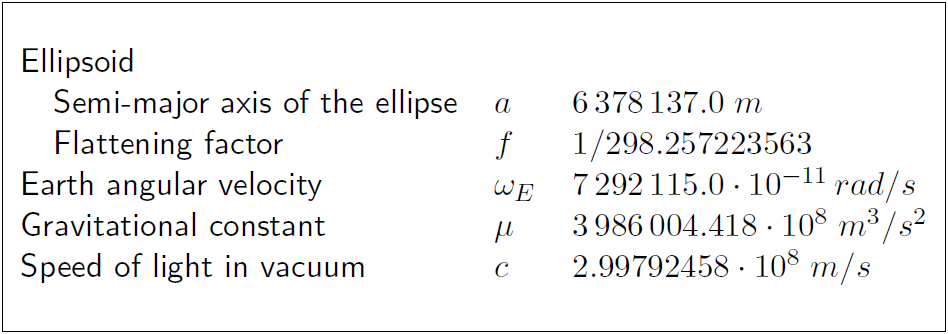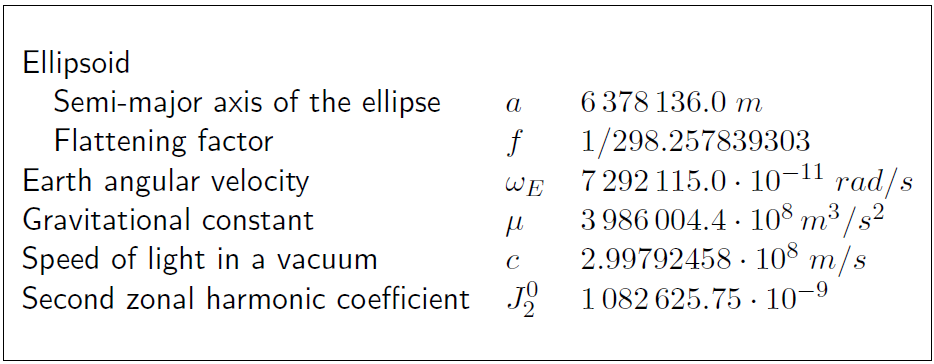If you wish to contribute or participate in the discussions about articles you are invited to contact the Editor

# Reference Frames in GNSSFundamentals
Title Reference Frames in GNSS
Author(s) J. Sanz Subirana, J.M. Juan Zornoza and M. Hernández-Pajares, Technical University of Catalonia, Spain.
Level Basic
Year of Publication 2011

Brief descriptions of GPS WGS-84, GLONASS PZ-90, Galileo GTRF and BeiDou Coordinate System (BDC) reference frames are provided as follows.

## GPS reference frame WGS-84

From 1987, GPS uses the World Geodetic System WGS-84, developed by the US Department of Defense (DoD) and now maintained by the US National Geospatial Intelligence Agency (NGA). WGS-84, is a unified terrestrial reference system for position and vector referencing [footnotes 1]. Indeed, the GPS broadcast ephemeris are linked to the position of the satellite antenna phase centre in the WGS-84 reference frame. Thus, the user receiver coordinates will be expressed in the same ECEF frame.

The initial implementation of WGS-84 was realized from a set of more than a thousand terrestrial sites, which coordinates were derived from Transit observations [footnotes 2]. Successive refinements (which also leaded to some adjustments of the fundamental constants), using more accurate coordinates of the monitor stations, approximate to some ITRS realizations. For instance, realizations WGS84(G730) [footnotes 3] and WGS84(G873) correspond to ITRF92 and ITRF94, respectively. The refined frame WGS84(G1150) was introduced in 2002, which agrees with ITRF2000 at the centimetre level. The most recent version is WGS-84 (G1762 ) and was implemented on October 2013 and is aligned to the International GNSS Service (IGS) realisation of the ITRF2008.

The parameters of the WGS-84 ellipsoid are given in the following table 1:Table 1: Ellipsoidal parameters WGS-84 (revised in 1997) and also in NGA Standard.

## GLONASS reference frame PZ-90

The GLONASS broadcast ephemeris are given in the Parametry Zemli 1990 (Parameters of the Earth 1990) (PZ-90) reference frame. As the WGS-84, this is an ECEF frame with a set of fundamental parameters associated (see table 2 from [GLONASS ICD, 2008]).

The determination of a set of parameters to transform the PZ-90 coordinates to the ITRF97 was the target of the International GLONASS Experiment (IGEX-98). [Boucher and Altamimi, 2001] presents a review of the IGEX-98 experiment and, as a conclusion, they suggest the following transformation [footnotes 4] from $(x,y,z)$ in PZ-90 to $(x',y',z')$ in WGS-84, with a meter level of accuracy.

$\left [ \begin{array}{c} x'\\ y'\\ z'\\ \end{array} \right ] = \left [ \begin{array}{c} x\\ y\\ z\\ \end{array} \right ] + \left [ \begin{array}{ccc} -3\,ppb & -353\,mas & -4\,mas\\ 353\,mas & -3\,ppb & 19\,mas\\ 4\,mas & -19\,mas & -3\,ppb\\ \end{array} \right ] \left [ \begin{array}{c} x\\ y\\ z\\ \end{array} \right ] + \left [ \begin{array}{c} 0.07\,m\\ -0.0\,m\\ -0.77\,m\\ \end{array} \right ] \qquad\mbox{(1)}$

Following the notation of equation (3) in Transformation between Terrestrial Frames:

$\left ( \begin{array}{c} x\\ y\\ z\\ \end{array} \right )_{_{TRF2}} = \left ( \begin{array}{c} x\\ y\\ z\\ \end{array} \right )_{_{TRF1}} + \left ( \begin{array}{c} T_1\\ T_2\\ T_3\\ \end{array} \right ) + \left ( \begin{array}{ccc} D & -R_3 & R_2\\ R_3 & D & -R_1\\ -R_2 & R_1 & D\\ \end{array} \right ) \left ( \begin{array}{c} x\\ y\\ z\\ \end{array} \right )_{_{TRF1}}$

the previous transformation (1) is defined by the parameters table:

According to the GLONASS modernisation plan, the ephemeris information implementing the PZ-90.11 reference system was updated on all operational GLONASS satellites starting from 3:00 pm on December 31, 2013. From this time on, the satellites are broadcasting in the PZ-90.11. This ECEF reference frame is an updated version of PZ-90, closest to the ITRF2000.

The transformation from PZ-90.11 to ITRF2008 contains only an origin shift vector, and no rotations nor scale factor, as it is shown in equation (2) [Revnivykh, 2007]

$\left [ \begin{array}{c} x\\ y\\ z\\ \end{array} \right ]_{ITRF2008} = \left [ \begin{array}{c} x\\ y\\ z\\ \end{array} \right ]_{PZ-90.11} + \left [ \begin{array}{r} 0.003\,m\,\pm0.002\,m\\ 0.001 \,m\,\pm0.002\,m\\ 0.001 \,m\,\pm0.002\,m\\ \end{array} \right ] \qquad\mbox{(2)}$

The parameters associated to the PZ-90 and PZ-90.11 are given in the next table 2 ([GLONASS ICD, 1998] and [GLONASS ICD, 2008]):Table 2: Ellipsoidal parameters of PZ-90 and PZ-90.11.

## Galileo Terrestrial Reference Frame (GTRF)

Next subsections provide an overview of the first realizations of Galileo Terrestrial Reference Frame, together with a description of the current applicable reference frame.

### First Galileo Reference Frames

A consortium named the Galileo Geodetic Service Provider (GGSP), consisting of seven institutions under the lead of GeoForschungsZentrum Potsdam, built up a prototype for the development of the Galileo Terrestrial Reference Frame (GTRF) and the establishment of a service with products and information for the potential users under an EC 6th framework contract.

Like GPS, Galileo established a dedicated terrestrial reference frame (GTRF) as an independent realization of the international terrestrial reference system ITRS based on the estimated coordinates for each one of the Galileo Sensor Station (GSS) sites. According to Galileo requirements the three-dimensional differences of the position compared to the most recent ITRF should not exceed 3 cm (2-sigma).

Operational GTRF includes all Galileo sensor stations (GSS) and selected stations of IGS. IGS stations are used for alignment to ITRF (as GSS are not a part of it) and for densification of the network to improve the accuracy of the results.

GTRF computation includes two parts: free network adjustment including all stations (GSS and selected IGS) and network alignment to ITRF using the IGS stations.

Under GGSP project, the four first realizations of GTRF were established:

The network included 131 IGS stations and 13 GESS (Galileo Experimental Sensor Stations for the GIOVE mission).

Already the initial realization of the GTRF called GTRF07v01 was in agreement with ITRF05 up to 0.9, 0.9 and 2.7 mm for North, East, and Up respectively.

The anticipated configuration of the operational GTRF network is presented in the figure below.

GTRF transformation parameters to ITRF2005 are reported below.

Solution GTRF08v01, epoch 07:241, used number of ITRF2005 sites: 103

### Current Galileo Terrestrial Reference Frame

Based on the outcomes of the previous section, the Galileo Terrestrial Reference Frame used by the Galileo system is described in the Galileo Open Service - Service Definition Document (SDD) . The Galileo navigation data (e.g. satellite ephemeris) is referenced to the GTRF. Accordingly, the user position coordinates derived from Galileo position solutions are referenced to GTRF.

Galileo GTRF is the independent and highly accurate realisation of the international Terrestrial Reference System based on the estimated coordinates of each one of the Galileo Sensor Station (GSS) sites. The Geodetic Reference Service Provider (GRSP) entity supports the Galileo Control Centre in realising the Galileo Terrestrial Reference Frame (GTRF).

At any time, the alignment between the GTRF and the latest physical realisation of the ITRF is such that the difference between the ITRF and the GTRF coordinates of the ITRF stations/markers used in the realisation of the GTRF is less than 3 cm (2σ). The GTRF is regularly aligned if new ITRF realisations are published. , Galileo OS user equipment needs to apply the appropriate valid transformation parameters between the latest ITRF and the desired reference frame.

## BeiDou Coordinate System (BDC)

The BeiDou Coordinate System (BDC) used to compute BeiDou navigation data is consistent with China Terrestrial Reference Frame (CGCS) 2000, which in turn is referred to ITRF97 with the epoch of 2000.0.

The main parameters of the reference ellipsoid are nearly the same as those defined by ITRS with the semi major axis (a), second degree harmonic coefficient (J2), and the mean angular velocity of the Earth (ω) the same as those of the ellipsoid of GRS1980. The gravitational mass (GM) constant adopts the value of WGS-84 (Wei, 2003). The flattening of the ellipsoid of BDC is f = 1/298.257222101.

Please find below the geodetic constants and parameters of Earth Ellipsoid CGCS2000: